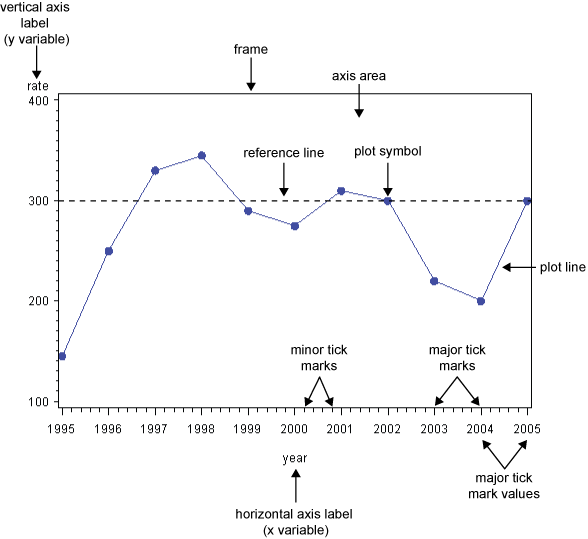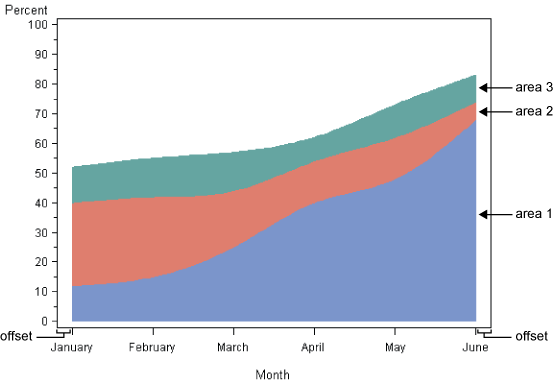The GPLOT Procedure

# Concepts

Some terms used with GPLOT procedure are illustrated in GPLOT Procedure Terms and Additional GPLOT Procedure Terms.The input data set that is used by the GPLOT procedure must contain at least one variable to plot on the horizontal axis and one variable to plot on the vertical axis. Typically, the horizontal axis shows an independent variable (time, for example), and the vertical axis shows a dependent variable (temperature, for example). With the exception of the Java and ActiveX device drivers, variables can be either character or numeric. For the ActiveX and Java device drivers, the horizontal axis can contain either character or numeric values, but the vertical axis can contain only numeric variables. Graphs are automatically scaled to the values of the character data or to include the values of numeric data, but you can control scaling with procedure options or with associated AXIS statements.

### Missing Values

If the value of either of the plot variables is missing, the GPLOT procedure does not include the observation in the plot. If you specify interpolation with a SYMBOL definition, the plot is not broken at the missing value. To break the plot line or area fill at the missing value, use the PLOT statement's SKIPMISS option. The SKIPMISS option is enabled only for JOIN interpolations.

### Values Out of Range

Data values can be excluded from a graph by restricting the range of axis values with the VAXIS= or HAXIS= options or with the ORDER= option in an AXIS statement. When an observation contains a value outside of the specified axis range, the GPLOT procedure excludes the observation from the plot and issues a message to the log.

If you specify interpolation with a SYMBOL definition, by default values outside of the axis range are excluded from interpolation calculations and as a result might change interpolated values for the plot. Values that are omitted from interpolation calculations have a particularly noticeable effect on the high-low interpolation methods: HILO, STD, and BOX. In addition, regression lines and confidence limits represent only part of the original data.

To specify that values out of range are included in the interpolation calculations, use the MODE= option in a SYMBOL statement. When MODE=INCLUDE, values that fall outside of the axis range are included in interpolation calculations but excluded from the plot. The default (MODE=EXCLUDE) omits observations that are outside of the axis range from interpolation calculations. See the MODE= option of the SYMBOL statement in SYMBOL Statement for details.

### Sorted Data

Data points are plotted in the order in which the observations are read from the data set. Therefore, if you use any type of interpolation that generates a line, sort your data by the horizontal axis variable.

### Logarithmic Axes

If your data contain logarithmic values or if the data values vary over a wide range or contain large values, you might want to specify a logarithmic axis for the horizontal or vertical axis. Logarithmic axes can be specified with the AXIS statement options LOGBASE= and LOGSTYLE=. See AXIS Statement for a complete discussion.Previous Page | Next Page | Top of Page